# Introduction to Fitting ASCII Data with Errors: Single-Component Source Models## Overview

#### Synopsis:

One-dimensional data from an ASCII file are empirically fit with polynomials of several orders. A parameter expression is defined to link the polynomial offset with one of the constants. The data and fits are plotted, and the plots are customized with matplotlib pyplot commands.

Last Update: 30 Mar 2022 - reviewed for CIAO 4.14, no content change; updated screen output

## Reading ASCII Data & Errors Into Sherpa

In this thread, we wish to fit 1-D data from the following ASCII data set:

```sherpa> !more data1.dat
0.5    1.6454   0.04114
1.5    1.7236   0.04114
2.5    1.9472   0.04114
3.5    2.2348   0.04114
4.5    2.6187   0.04114
5.5    2.8642   0.04114
6.5    3.1263   0.04114
7.5    3.2073   0.04114
8.5    3.2852   0.04114
9.5    3.3092   0.04114
10.5   3.4496   0.04114
```

This data set is input into Sherpa using the load_data() function, with the first argument setting the data set ID to 1 (default), the second indicating the filename, and the third indicating the number of columns to read. The first and second columns are read in as x and y(x), and the third column is read in as the statistical errors:

```sherpa> load_data(1, "data1.dat", 3)
sherpa> show_data()
Data Set: 1
Filter: 0.5000-10.5000 x
name      = data1.dat
x         = Float64
y         = Float64
staterror = Float64
syserror  = None

sherpa> get_indep()
(array([  0.5,   1.5,   2.5,   3.5,   4.5,   5.5,   6.5,   7.5,   8.5,
9.5,  10.5]),)

sherpa> get_staterror()
array([ 0.04114,  0.04114,  0.04114,  0.04114,  0.04114,  0.04114,
0.04114,  0.04114,  0.04114,  0.04114,  0.04114])
```

In the following example, data2.dat represents an ASCII file with one line of header information (column names), and data3.dat is a file with three lines of header information (the last line containing column names):

```sherpa>  load_data(2, "data2.dat", 3)
sherpa>  show_data(2)
Data Set: 2
Filter: 0.5000-10.5000 x
name      = data2.dat
x         = Float64
y         = Float64
staterror = Float64
syserror  = None

sherpa>  !dmlist data2.dat data,clean
#  xval                 func                 err
0.50              2.46020             0.061510
1.50              2.46020             0.061510
2.50              2.63730             0.061510
3.50              2.93930             0.061510
4.50              3.26280             0.061510
5.50              3.41310             0.061510
6.50              3.49990             0.061510
7.50              3.60380             0.061510
8.50              3.63740             0.061510
9.50              3.87280             0.061510
10.50              4.33250             0.061510

sherpa> show_data(3)
Data Set: 3
Filter: 21.0000-24.0000 x
name      = data3.dat[opt skip=2]
x         = Float64
y         = Float64
staterror = Float64
syserror  = None

sherpa> !dmlist "data3.dat[opt skip=2]" data,clean
#  xval                 func                 err
21.0                41.30                21.80
22.0                41.10                20.20
23.0                43.80                17.30
24.0                12.30                11.10
sherpa> !more data3.dat
# data3.dat
#
# xval func err
21.0  41.3  21.8
22.0  41.1  20.2
23.0  43.8  17.3
24.0  12.3  11.1
```

As shown, the first two lines of the header in data3.dat were skipped with the optional argument supplied to load_data.

For more information on using ASCII-format files in Sherpa, see the Sherpa "Command Comparison" FAQ #3. To learn the details of the CIAO DM ASCII kernel, see the dmascii ahelp file.

## Plotting Data

Now the data set may be plotted:

```sherpa> plot_data()
```

The CIAO software uses the Python plotting application matplotlib. matplotlib's pyplot plotting can be used within Sherpa to modify the appearance of a plot. The plt.xlabel() and plt.ylabel() commands are used to add axis labels:

```sherpa> plt.xlabel("X = Off-Axis (arcmin)")

sherpa> plt.ylabel("F(X) = SNR")
```

Figure 1 shows the resulting plot.

### Figure 1: Data plotted with errors (Python)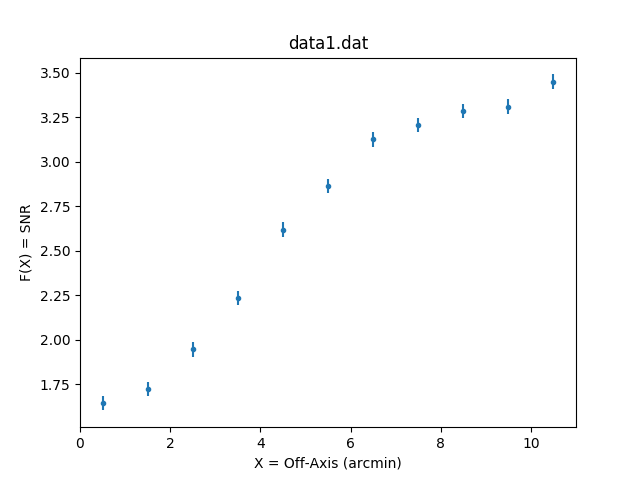## Establishing a Model Component

A polynomial model will be used to fit the data. The Sherpa model name for a 1-D polynomial function is polynom1d.

The model is established using the set_source() function, specifying the model name for the session. Here, the generic polynom1d model is named model1:

```sherpa> set_source(polynom1d.model1)
```

The get_model_type() and show_model() commands may be used to examine the details of the established model. The initial parameter settings—thawed or frozen, initial values, minimum and maximum allowed values—are listed:

```sherpa> get_model_type("model1")
'polynom1d'

sherpa> show_model()
Model: 1
polynom1d.model1
Param        Type          Value          Min          Max      Units
-----        ----          -----          ---          ---      -----
model1.c0    thawed            1 -3.40282e+38  3.40282e+38
model1.c1    frozen            0 -3.40282e+38  3.40282e+38
model1.c2    frozen            0 -3.40282e+38  3.40282e+38
model1.c3    frozen            0 -3.40282e+38  3.40282e+38
model1.c4    frozen            0 -3.40282e+38  3.40282e+38
model1.c5    frozen            0 -3.40282e+38  3.40282e+38
model1.c6    frozen            0 -3.40282e+38  3.40282e+38
model1.c7    frozen            0 -3.40282e+38  3.40282e+38
model1.c8    frozen            0 -3.40282e+38  3.40282e+38
model1.offset frozen            0 -3.40282e+38  3.40282e+38

```

## Viewing Method & Statistic Settings

We use Sherpa's default optimization method and statistics for these polynomial fits. To view the current method and statistics settings:

```sherpa> show_method()
Optimization Method: LevMar
name     = levmar
ftol     = 1.1920928955078125e-07
xtol     = 1.1920928955078125e-07
gtol     = 1.1920928955078125e-07
maxfev   = None
epsfcn   = 1.1920928955078125e-07
factor   = 100.0
numcores = 1
verbose  = 0

sherpa> show_stat()
Statistic: Chi2Gehrels
Chi Squared with Gehrels variance.

The variance is estimated from the number of counts in each bin,
but unlike `Chi2DataVar`, the Gaussian approximation is not
used. This makes it more-suitable for use with low-count data.

The standard deviation for each bin is calculated using the
approximation from _:

sigma(i,S) = 1 + sqrt(N(i,s) + 0.75)

where the higher-order terms have been dropped. This is accurate
to approximately one percent. For data where the background has
not been subtracted then the error term is:

sigma(i) = sigma(i,S)

whereas with background subtraction,

sigma(i)^2 = sigma(i,S)^2 + [A(S)/A(B)]^2 sigma(i,B)^2

A(B) is the off-source "area", which could be
the size of the region from which the background is extracted, or
the length of a background time segment, or a product of the two,
etc.; and A(S) is the on-source "area". These terms may be defined
for a particular type of data: for example, PHA data sets A(B) to
`BACKSCAL * EXPOSURE` from the background data set and A(S) to
`BACKSCAL * EXPOSURE` from the source data set.

--------
Chi2DataVar, Chi2ModVar, Chi2XspecVar

Notes
-----
The accuracy of the error term when the background has been
subtracted has not been determined. A preferable approach to
background subtraction is to model the background as well as the
source signal.

References
----------

..  "Confidence limits for small numbers of events in
astrophysical data", Gehrels, N. 1986, ApJ, vol 303,
p. 336-346.
```

Further details about the Levenberg-Marquardt optimization method are available by typing:

```sherpa> ahelp "levmar"
```

Further details about the χ2 Gehrels statistic are available by typing:

```sherpa> ahelp "chi2gehrels"
```

## Thawing Model Parameters & Fitting

To start, we wish to fit these data with a first-order polynomial. The c1 parameter of model1 needs to be thawed so that it will be allowed to vary during the fit:

```sherpa> thaw(model1.c1)
sherpa> show_model()
Model: 1
polynom1d.model1
Param        Type          Value          Min          Max      Units
-----        ----          -----          ---          ---      -----
model1.c0    thawed            1 -3.40282e+38  3.40282e+38
model1.c1    thawed            0 -3.40282e+38  3.40282e+38
model1.c2    frozen            0 -3.40282e+38  3.40282e+38
model1.c3    frozen            0 -3.40282e+38  3.40282e+38
model1.c4    frozen            0 -3.40282e+38  3.40282e+38
model1.c5    frozen            0 -3.40282e+38  3.40282e+38
model1.c6    frozen            0 -3.40282e+38  3.40282e+38
model1.c7    frozen            0 -3.40282e+38  3.40282e+38
model1.c8    frozen            0 -3.40282e+38  3.40282e+38
model1.offset frozen            0 -3.40282e+38  3.40282e+38
```

We can use the Sherpa guess command to guess the initial parameter values and ranges for the model parameters (as model parameter values are not automatically guessed in Sherpa as they were in previous versions of the software). To have Sherpa automatically query for the initial parameter values when a model is established, set 'paramprompt(True)' (it is 'False' by default).

```sherpa> guess(model1)

sherpa> show_model()
Model: 1
polynom1d.model1
Param        Type          Value          Min          Max      Units
-----        ----          -----          ---          ---      -----
model1.c0    thawed       2.5475      -1.6454       3.4496
model1.c1    thawed            0      -18.042       18.042
model1.c2    frozen            0      -1.8042       1.8042
model1.c3    frozen            0      -1.6454       3.4496
model1.c4    frozen            0      -1.6454       3.4496
model1.c5    frozen            0      -1.6454       3.4496
model1.c6    frozen            0      -1.6454       3.4496
model1.c7    frozen            0      -1.6454       3.4496
model1.c8    frozen            0      -1.6454       3.4496
model1.offset frozen            0 -3.40282e+38  3.40282e+38
```Tip

The guess command makes an initial guess at parameter values to ensure convergence, but it is always a good idea to check that the initial range of values (soft limits) is sensible for the data being fit.

The data set is then fit using the default optimization method and statistic:

```sherpa> fit()
Dataset               = 1
Method                = levmar
Statistic             = chi2
Initial fit statistic = 2815.14
Final fit statistic   = 151.827 at function evaluation 6
Data points           = 11
Degrees of freedom    = 9
Probability [Q-value] = 3.68798e-28
Reduced statistic     = 16.8697
Change in statistic   = 2663.31
model1.c0      1.58227      +/- 0.0248858
model1.c1      0.198455     +/- 0.00392255
```

The fit information includes the χ2 statistic value for chi2gehrels, goodness-of-fit and reduced χ2 along with the best-fit parameter values.

To plot the fit model and the data, use the plot_fit() function:

By default, the fit is plotted as a red line over the data, as shown in Figure 2. Clearly a first-order polynomial does not give a good fit to the data set.

### Figure 2: First-order polynomial fit to the data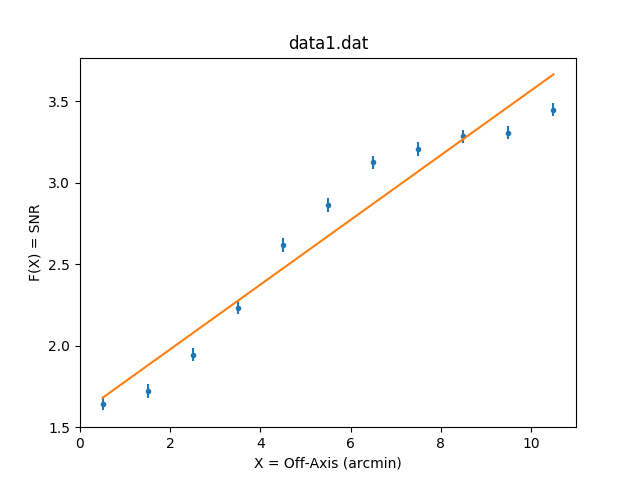### Figure 2: First-order polynomial fit to the data

The c2 parameter of model1 is thawed so that a second-order polynomial may be fit to the data:

```sherpa> thaw(model1.c2)
sherpa> guess(model1)
sherpa> fit()
Dataset               = 1
Method                = levmar
Statistic             = chi2
Initial fit statistic = 2815.14
Final fit statistic   = 59.0027 at function evaluation 8
Data points           = 11
Degrees of freedom    = 8
Probability [Q-value] = 7.31065e-10
Reduced statistic     = 7.37534
Change in statistic   = 2756.14
model1.c0      1.30826      +/- 0.0377915
model1.c1      0.347303     +/- 0.0159396
model1.c2      -0.0135317   +/- 0.0014045

```

Plot the fit and the data:

```sherpa> plot_fit()
sherpa> plt.xlabel("X = Off-Axis (arcmin)")
sherpa> plt.ylabel("F(X) = SNR")
```

Figure 3 shows the plot of the second-order polynomial fit.

### Figure 3: Second-order polynomial fit to the data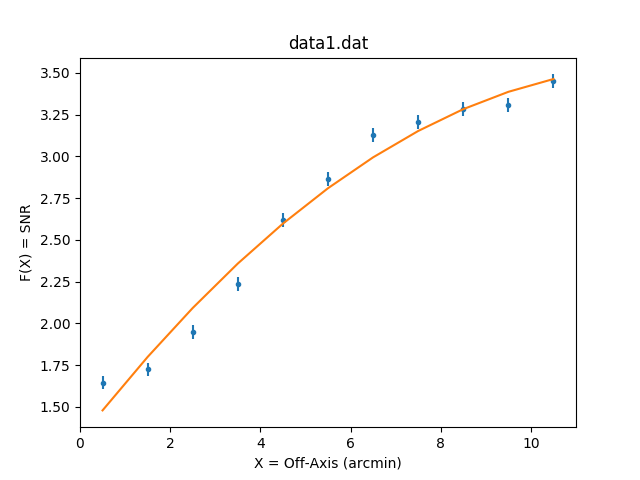### Figure 3: Second-order polynomial fit to the data

Finally, the data are fit with a third-order polynomial. The c3 parameter of model1 is thawed, then the data is fit again and plotted:

```sherpa> thaw(model1.c3)
sherpa> guess(model1)
sherpa> fit()
Dataset               = 1
Method                = levmar
Statistic             = chi2
Initial fit statistic = 2815.14
Final fit statistic   = 30.8491 at function evaluation 10
Data points           = 11
Degrees of freedom    = 7
Probability [Q-value] = 6.62869e-05
Reduced statistic     = 4.40701
Change in statistic   = 2784.29
model1.c0      1.49843      +/- 0.0520846
model1.c1      0.1447       +/- 0.0413773
model1.c2      0.0322936    +/- 0.00874997
model1.c3      -0.00277729  +/- 0.000523425

sherpa> plot_fit()
sherpa> plt.xlabel("X = Off-Axis (arcmin)")
sherpa> plt.ylabel("F(X) = SNR")
```

Figure 4 shows the plot of the third-order polynomial fit together with the data.

### Figure 4: Third-order polynomial fit to the data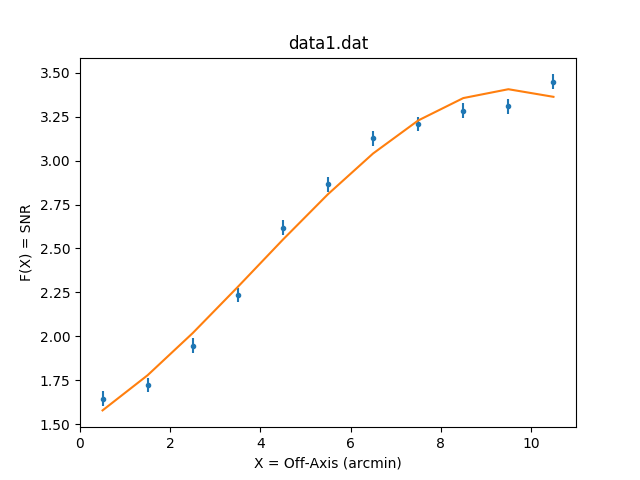## Examining Fit Results

The best fit model with the data and residuals may be plotted in the same window:

```sherpa> plot_fit_resid()
```

Various modifications may be made to the plots:

```# set current plot to the lower residual plot
sherpa> fig = plt.gcf()
sherpa> plt.sca(fig.axes)

# Label the x-axis; add minor tickmarks
sherpa> plt.xlabel("X = Off-Axis (arcmin)")
sherpa> plt.minorticks_on()
sherpa> plt.tick_params(which="both",direction="in",bottom=True,top=True,right=True,left=True)

# set current plot to the upper fit plot; label the y-axis; add minor tickmarks
sherpa> plt.sca(fig.axes)
sherpa> plt.ylabel("F(X) = SNR")
sherpa> plt.minorticks_on()
sherpa> plt.tick_params(which="both",direction="in",bottom=True,top=True,right=True,left=True)

# Change the title; adjust the space between the two plots
sherpa> plt.title("ACIS 25000 Counts Per Chip")

# Add a label that describes the third-order polynomial fit
sherpa> plt.text(x=3.0,y=1.7,s="\$F(X)=(1.4984)+(0.1447)X+(0.0323)X^2+(-0.0028)X^3\$",fontsize=8)
```

The "#" sign indicates a comment and will be ignored if entered on the command line.

### Figure 5: Plotting the fit and residuals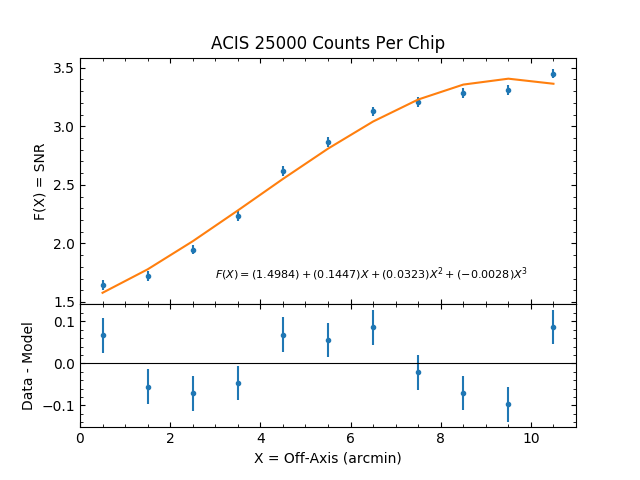### Figure 5: Plotting the fit and residuals

Use covariance (covar()) or confidence (conf()) to estimate the confidence intervals for the thawed parameters. By default, the 1σ upper and lower bounds are returned:

```sherpa> covar()
Dataset               = 1
Confidence Method     = covariance
Fitting Method        = levmar
Statistic             = chi2gehrels
covariance 1-sigma (68.2689%) bounds:
Param            Best-Fit  Lower Bound  Upper Bound
-----            --------  -----------  -----------
model1.c0         1.49843   -0.0520846    0.0520846
model1.c1          0.1447   -0.0413773    0.0413773
model1.c2       0.0322936  -0.00874997   0.00874997
model1.c3     -0.00277729 -0.000523425  0.000523425

```

In CIAO 3.4, the GOODNESS command was used to get the χ2 goodness-of-fit. This information is now reported with the best-fit values after a fit, as well as with the CIAO 4.x equivalent to the 3.4 GOODNESS command, calc_stat_info and get_stat_info. These functions, along with the show_fit and get_fit_results commands, allow access to this information after the fit has been performed.

For the final fit (third-order polynomial), the results are:

```Final fit statistic = 30.8491 at function evaluation 10
Data points = 11
Degrees of freedom = 7
Probability [Q-value] = 6.62869e-05
Reduced statistic  = 4.40701
```

Instead of empirically fitting a polynomial to the data as before, we now wish to fit a first order polynomial such that the offset constant parameter is the following product:

```  offset = 4.3979 * c1
```

where c1 is the first order coefficient and 4.3979 = log10(25000).

First, a new polynom1d model is established and is named model2:

```sherpa> set_source(polynom1d.model2)
```

For a third-order polynomial fit, thaw the first three constant parameters of model2:

```sherpa> thaw(model2.c1,model2.c2,model2.c3)
```

The offset parameter of model2 is linked to the value of the c1 parameter:

```sherpa> model2.offset = (model2.c1)*(4.3979)
```

The resulting source model expression is:

```sherpa> show_model()
Model: 1
polynom1d.model2
Param        Type          Value          Min          Max      Units
-----        ----          -----          ---          ---      -----
model2.c0    thawed            1 -3.40282e+38  3.40282e+38
model2.c1    thawed            0 -3.40282e+38  3.40282e+38
model2.c2    thawed            0 -3.40282e+38  3.40282e+38
model2.c3    thawed            0 -3.40282e+38  3.40282e+38
model2.c4    frozen            0 -3.40282e+38  3.40282e+38
model2.c5    frozen            0 -3.40282e+38  3.40282e+38
model2.c6    frozen            0 -3.40282e+38  3.40282e+38
model2.c7    frozen            0 -3.40282e+38  3.40282e+38
model2.c8    frozen            0 -3.40282e+38  3.40282e+38
model2.offset linked            0 expr: (model2.c1 * 4.3979)

sherpa> print(get_model().offset)
val         = 0.0
min         = -3.40282346639e+38
max         = 3.40282346639e+38
units       =
frozen      = True
default_val = 0.0
default_min = -3.40282346639e+38
default_max = 3.40282346639e+38
```

Here the details of the link of the model2.offset are shown along with the initial settings.

The data set is then fit:

```sherpa> fit()
Dataset               = 1
Method                = levmar
Statistic             = chi2gehrels
Initial fit statistic = 20919.3
Final fit statistic   = 30.8491 at function evaluation 25
Data points           = 11
Degrees of freedom    = 7
Probability [Q-value] = 6.62869e-05
Reduced statistic     = 4.40701
Change in statistic   = 20888.4
model2.c0      1.64339      +/- 0.0257821
model2.c1      0.193666     +/- 0.0361465
model2.c2      0.0251972    +/- 0.00869733
model2.c3      -0.00277729  +/- 0.000523425

```

Plot the best fit model and add axis labels:

```sherpa> plot_fit()
sherpa> plt.xlabel("X = Off-Axis (arcmin)")
sherpa> plt.ylabel("F(X) = SNR")
```

Figure 6 shows the resulting plot.

### Figure 6: Fitting using linked model parameters### Figure 6: Fitting using linked model parameters

To compare the fitting results obtained using model2 to those obtained using model1:

```sherpa> print(model1)
polynom1d.model1
Param        Type          Value          Min          Max      Units
-----        ----          -----          ---          ---      -----
model1.c0    thawed      1.49843      -1.6454       3.4496
model1.c1    thawed       0.1447      -18.042       18.042
model1.c2    thawed    0.0322936      -1.8042       1.8042
model1.c3    thawed  -0.00277729      -1.6454       3.4496
model1.c4    frozen            0 -3.40282e+38  3.40282e+38
model1.c5    frozen            0 -3.40282e+38  3.40282e+38
model1.c6    frozen            0 -3.40282e+38  3.40282e+38
model1.c7    frozen            0 -3.40282e+38  3.40282e+38
model1.c8    frozen            0 -3.40282e+38  3.40282e+38
model1.offset frozen            0 -3.40282e+38  3.40282e+38

sherpa> print(model2)
polynom1d.model2
Param        Type          Value          Min          Max      Units
-----        ----          -----          ---          ---      -----
model2.c0    thawed      1.64339 -3.40282e+38  3.40282e+38
model2.c1    thawed     0.193666 -3.40282e+38  3.40282e+38
model2.c2    thawed    0.0251972 -3.40282e+38  3.40282e+38
model2.c3    thawed  -0.00277729 -3.40282e+38  3.40282e+38
model2.c4    frozen            0 -3.40282e+38  3.40282e+38
model2.c5    frozen            0 -3.40282e+38  3.40282e+38
model2.c6    frozen            0 -3.40282e+38  3.40282e+38
model2.c7    frozen            0 -3.40282e+38  3.40282e+38
model2.c8    frozen            0 -3.40282e+38  3.40282e+38
model2.offset linked     0.851724 expr: (model2.c1 * 4.3979)

```

Again, the χ2 goodness-of-fit was reported with the fit results:

```Final fit statistic value = 30.8491 at function evaluation 25
Data points = 11
Degrees of freedom = 7
Probability [Q-value] = 6.62869e-05
Reduced statistic  = 4.40701
```

## Independently Fitting a Second Data Set

Finally, we fit a different data set with a third-order polynomial.

This data set and errors are read and loaded into Sherpa as data set with id="2" so that the previous data set (with the default id="1") isn't overwritten:

```sherpa> !more data2.dat
0.5    2.4602   0.06151
1.5    2.4602   0.06151
2.5    2.6373   0.06151
3.5    2.9393   0.06151
4.5    3.2628   0.06151
5.5    3.4131   0.06151
6.5    3.4999   0.06151
7.5    3.6038   0.06151
8.5    3.6374   0.06151
9.5    3.8728   0.06151
10.5   4.3325   0.06151

sherpa> show_data(2)

Data Set: 2
Filter: 0.5000-10.5000 x
name      = data2.dat
x         = Float64
y         = Float64
staterror = Float64
syserror  = None
```

Data Set id=2 may now be plotted. Note that you need to specify the id in the plot_data() function:

```sherpa> plot_data(2)
sherpa> plt.xlabel("X = Off-Axis (arcmin)")
sherpa> plt.ylabel("F(X) = SNR")
```

Another polynom1d model—model3—is established and the first three constants are thawed:

```sherpa> set_source(2,polynom1d.model3)
sherpa> thaw(model3.c1,model3.c2,model3.c3)
sherpa> guess(model3)
```

Data Set id=2 is then fit with the model3. Note that id has been explicitly stated in the fit() function:

```sherpa> fit(2)
Dataset               = 2
Method                = levmar
Statistic             = chi2
Initial fit statistic = 2534.99
Final fit statistic   = 35.5324 at function evaluation 10
Data points           = 11
Degrees of freedom    = 7
Probability [Q-value] = 8.88089e-06
Reduced statistic     = 5.07605
Change in statistic   = 2499.46
model3.c0      2.19527      +/- 0.0778737
model3.c1      0.286636     +/- 0.0618648
model3.c2      -0.0234649   +/- 0.0130824
model3.c3      0.0013769    +/- 0.000782593

```

And the best fit model is plotted:

```sherpa> plot_fit(2)
sherpa> plt.xlabel("X = Off-Axis (arcmin)")
sherpa> plt.ylabel("F(X) = SNR")
```

This data set may need another order in the polynomial, so we free model3.c4 and fit again:

```sherpa> thaw(model3.c4)
sherpa> guess(model3)
sherpa> fit(2)
Dataset               = 2
Method                = levmar
Statistic             = chi2
Initial fit statistic = 2534.99
Final fit statistic   = 1.18643 at function evaluation 12
Data points           = 11
Degrees of freedom    = 6
qProbability [Q-value] = 0.97755
Reduced statistic     = 0.197739
Change in statistic   = 2533.81
model3.c0      2.60526      +/- 0.104683
model3.c1      -0.407013    +/- 0.133552
model3.c2      0.254528     +/- 0.0492057
model3.c3      -0.0377019   +/- 0.0067139
model3.c4      0.00177631   +/- 0.000303097

sherpa> plot_fit(2)
sherpa> plt.xlabel("X = Off-Axis (arcmin)")
sherpa> plt.ylabel("F(X) = SNR")
```

Figure 7 shows the resulting plot.

### Figure 7: Fitting a second data set with a fourth-order polynomial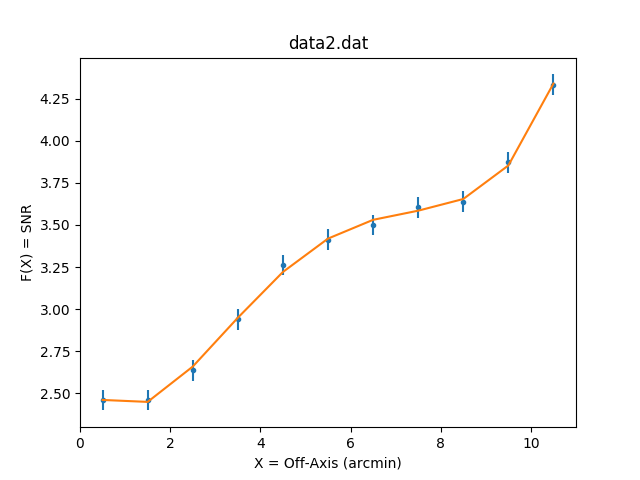## Checking Sherpa Session Status

A variety of information about the final status of this Sherpa session may be obtained:

```# method and statistic setting
sherpa> show_stat()
Statistic: Chi2Gehrels
Chi Squared with Gehrels variance.

The variance is estimated from the number of counts in each bin,
but unlike `Chi2DataVar`, the Gaussian approximation is not
used. This makes it more-suitable for use with low-count data.

The standard deviation for each bin is calculated using the
approximation from _:

sigma(i,S) = 1 + sqrt(N(i,s) + 0.75)

where the higher-order terms have been dropped. This is accurate
to approximately one percent. For data where the background has
not been subtracted then the error term is:

sigma(i) = sigma(i,S)

whereas with background subtraction,

sigma(i)^2 = sigma(i,S)^2 + [A(S)/A(B)]^2 sigma(i,B)^2

A(B) is the off-source "area", which could be
the size of the region from which the background is extracted, or
the length of a background time segment, or a product of the two,
etc.; and A(S) is the on-source "area". These terms may be defined
for a particular type of data: for example, PHA data sets A(B) to
`BACKSCAL * EXPOSURE` from the background data set and A(S) to
`BACKSCAL * EXPOSURE` from the source data set.

--------
Chi2DataVar, Chi2ModVar, Chi2XspecVar

Notes
-----
The accuracy of the error term when the background has been
subtracted has not been determined. A preferable approach to
background subtraction is to model the background as well as the
source signal.

References
----------

..  "Confidence limits for small numbers of events in
astrophysical data", Gehrels, N. 1986, ApJ, vol 303,
p. 336-346.

# observed counts in each data set
sherpa> calc_data_sum(id=1)
29.4115

sherpa> calc_data_sum(id=2)
36.119299999999996

# what types of models are defined
sherpa> get_model_type("model1")
'polynom1d'
sherpa> get_model_type("model2")
'polynom1d'
sherpa> get_model_type("model3")
'polynom1d'

# final fit values for the source models
sherpa> show_model(1)
Model: 1
polynom1d.model2
Param        Type          Value          Min          Max      Units
-----        ----          -----          ---          ---      -----
model2.c0    thawed      1.64339 -3.40282e+38  3.40282e+38
model2.c1    thawed     0.193666 -3.40282e+38  3.40282e+38
model2.c2    thawed    0.0251972 -3.40282e+38  3.40282e+38
model2.c3    thawed  -0.00277729 -3.40282e+38  3.40282e+38
model2.c4    frozen            0 -3.40282e+38  3.40282e+38
model2.c5    frozen            0 -3.40282e+38  3.40282e+38
model2.c6    frozen            0 -3.40282e+38  3.40282e+38
model2.c7    frozen            0 -3.40282e+38  3.40282e+38
model2.c8    frozen            0 -3.40282e+38  3.40282e+38
model2.offset linked     0.851724 expr: (model2.c1 * 4.3979)

sherpa> show_model(2)
Model: 2
polynom1d.model3
Param        Type          Value          Min          Max      Units
-----        ----          -----          ---          ---      -----
model3.c0    thawed      2.60526      -1.6454       3.4496
model3.c1    thawed    -0.407013      -18.042       18.042
model3.c2    thawed     0.254528      -1.8042       1.8042
model3.c3    thawed   -0.0377019      -1.6454       3.4496
model3.c4    thawed   0.00177631      -1.6454       3.4496
model3.c5    frozen            0 -3.40282e+38  3.40282e+38
model3.c6    frozen            0 -3.40282e+38  3.40282e+38
model3.c7    frozen            0 -3.40282e+38  3.40282e+38
model3.c8    frozen            0 -3.40282e+38  3.40282e+38
model3.offset frozen            0 -3.40282e+38  3.40282e+38
```

## Exiting Sherpa

To exit the current Sherpa session:

```sherpa> quit()
```

## Scripting It

The file fit.py is a Python script which performs the primary commands used above; it can be executed by typing %run -i fit.py on the Sherpa command line.

The Sherpa script command may be used to save everything typed on the command line in a Sherpa session:

```sherpa> script(filename="sherpa.log", clobber=False)
```

(Note that restoring a Sherpa session from such a file could be problematic since it may include syntax errors, unwanted fitting trials, et cetera.)

## History

 14 Nov 2007 rewritten for CIAO 4.0 Beta 3 09 Dec 2008 figures placed inline with text 12 Dec 2008 get_indep and get_staterror are available in Sherpa 4.1 16 Feb 2009 example of guess functionality added 22 Apr 2009 load_data supports DM ASCII syntax as of the CIAO 4.1.2 release 29 Apr 2009 new script command is available with CIAO 4.1.2 17 Dec 2009 updated for CIAO 4.2 13 Jul 2010 updated for CIAO 4.2 Sherpa v2: removal of S-Lang version of thread. 15 Dec 2010 updated for CIAO 4.3: the new calc_stat_info and get_stat_info commands are available for accessing goodness-of-fit information after a fit, similar to the CIAO 3.4 GOODNESS command 15 Dec 2011 reviewed for CIAO 4.4 (no changes) 30 Aug 2013 Replace set_axis_tickmark which is only in the advanced chips UI, with the standard set_axis command. 03 Dec 2013 reviewed for CIAO 4.6 (no changes) 03 Dec 2014 updated for CIAO 4.7, no content changes 01 Dec 2015 updated for CIAO 4.8, no content change 31 Oct 2016 reviewed for CIAO 4.9, no content change; updated screen output 10 Apr 2018 reviewed for CIAO 4.10, no content change 03 Dec 2018 reviewed for CIAO 4.11; updated screen output 10 Dec 2019 reviewed for CIAO 4.12; updated plotting commands and figures to use matplotlib 30 Mar 2022 reviewed for CIAO 4.14, no content change; updated screen output